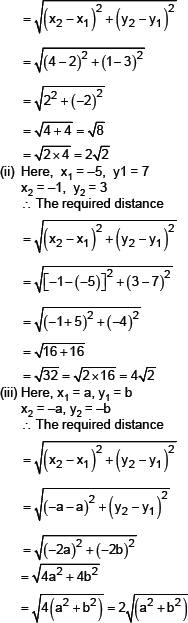#### EXERCISE

###### Question 1 :
Q.1.   Find the distance between the following pairs of points:
(i) (2, 3), (4, 1)
(ii) (–5, 7), (–1, 3)
(iii) (a, b), (–a, –b)
###### Formulae used :

Distance Formula to find distance between two points (x1,y1) and (x2,y2) is D = √[(x2 – x1)2 + (y2 – y1)2 ]. The distance formula to find the distance of a point P(x, y) from the origin O(0,0) is D = √((x2 + y2).

###### Solution :
Sol. (i) Here x1 = 2, y1 = 3, x2 = 4 and y2 = 1
∴ The required distance###### Practice Questions
Q.1.   Find the distance between the following pairs of points:
(i) (2, 3), (4, 1)
(ii) (–5, 7), (–1, 3)
(iii) (a, b), (–a, –b)
Q.1.   Find the distance between the following pairs of points:
(i) (2, 3), (4, 1)
(ii) (–5, 7), (–1, 3)
(iii) (a, b), (–a, –b)
Q.1.   Find the distance between the following pairs of points:
(i) (2, 3), (4, 1)
(ii) (–5, 7), (–1, 3)
(iii) (a, b), (–a, –b)